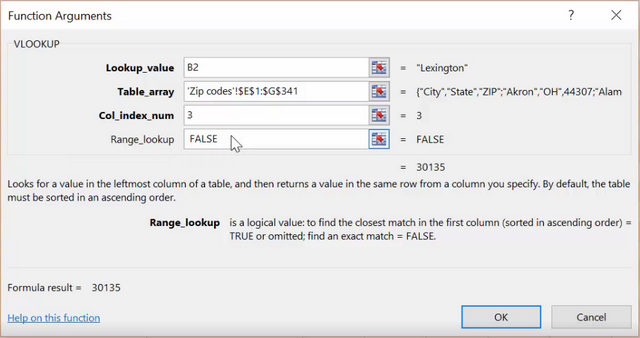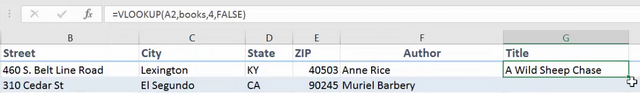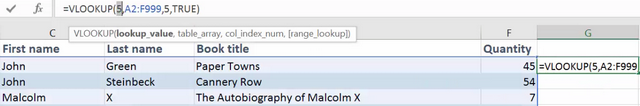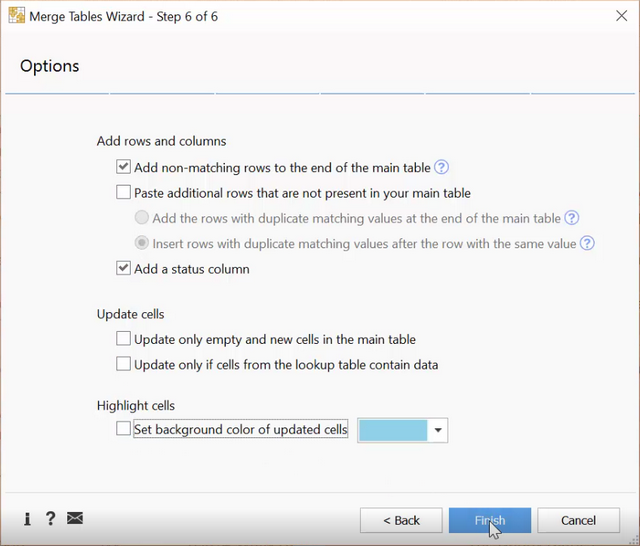# Excel VLOOKUP explained - how to video

If the VLOOKUP function in Excel ever intimidated you, watch this video to understand its structure and learn how to do vlookup yourself.

## What is VLOOKUP: video transcript

In this video we will explore the Vlookup function. Vertical lookup is what you need when you have interrelated information in different Excel tables. Here is a quick example: you have a list of addresses and cities, and you can quickly pull the corresponding ZIP codes from the reference table.

### The structure of the VLOOKUP function in Excel

Let's look at the structure of the formula to get a better understanding of what it needs to work. We can specify four arguments:1. The Lookup value is the common record you can see in both lists: the cities.
• You can enter text here, for example the name of the city, just make sure you use quotes around it.
• If your key value is a number, e.g. if you wanted to find the city name by the ZIP code, you'd simply enter it as it is.
• Perhaps the most valuable option of all is the possibility to include a cell reference here. If we point to a cell with the key value, we'll be able to quickly replicate the formula for all similar cells below.
2. Table_array is the reference table you are looking at. As the formula will check the leftmost column for the key values, it must always come first. And the values we want to get must be somewhere to the right. To use the formula for other cells, it is important to make this range fixed, or absolute. To do this, add the dollar sign before the columns and rows or press F4.
3. We need to enter the number of the column with values we want to get. To understand what this number is, open your lookup table and count columns from left to right, the key column being your first one. The one with the ZIP codes is the third here.
4. Finally, write FALSE in the last field if you are looking for an exact match. Even though this argument is optional, remember to include it to avoid unexpected results.

Let's take a quick look at the formula to read it: find this city in the first column of this range and return the corresponding Zip Code from the third column there when you find an exact match.

Now that you know the basics, let's try a different example and consider some more details.

### How to use Vlookup in Excel

Here we have the book IDs and authors and we need to look up the book title in another sheet. There is one prerequisite that will make your work a lot easier: select the lookup values starting with your key column and enter a name for the range into this box.

Now you are ready to enter the formula:

`=VLOOKUP(A2, Books, 4, FALSE)`Since we need to get book titles for all the IDs we have here, begin with a reference to the cell with the key value: A2. Now you could select the range in your lookup table and make it absolute by adding dollar signs, or simply enter the name you created for it. Add a comma, and count the number of the column with the book names. Remember to enter False and close parentheses.

Copy the formula down to get the results for all the cells below. Notice how you get N/A error for those key records that are missing in the lookup table? You can replace it with anything, from a blank to "Not Found" text by wrapping the VLOOKUP formula into IFERROR function and adding the text you want to see in its place:

`=IFERROR(VLOOKUP(A2, Books, 4, FALSE), "")`

Now it says if there is an error as a result of the formula, then keep the cell empty.

### Vlookup TRUE or FALSE: searching for an approximate match in the key columns

Let's consider one more example for those cases when you are not looking for an exact match. It can be especially convenient when you work with numbers.

Say, I want to find a book with the rating as close to 5 as possible.

• First of all, I'll need to sort my range by the key column in ascending order (a to z).
• Then enter VLOOKUP formula:

`=VLOOKUP(5,E2:F999,2,TRUE) `Add my lookup rating "5", a reference to the range, the number of the column with the books, and enter TRUE for the last argument or skip it altogether.

Now the formula is looking for the next largest value.

### How to do VLOOKUP in excel without formulas

If you are short of time, you can skip the formulas and get vlookup help from our Merge Tables Wizard add-in for Excel. Once you install it, you'll find it under Ablebits Data tab. Let me quickly go over the simple steps to follow:

1. First you select your main table to update
2. Then choose the lookup table
3. Pick the key columns, their order is not important and you can check the combination of any values
4. Then choose what columns you want to update in your main table
5. And which new ones to add
6. There are also additional options: add new key values if they are not in your main table, handle duplicates, color the changes, and some more. Click Finish to get the merge results.It's a great assistant if you often update tables, and you can get it with a discount if you use the coupon code Preview.

I hope you have a better understanding of how VLOOKUP works in Excel. If you have any questions, please share them as comments, we'll do our best to help you.